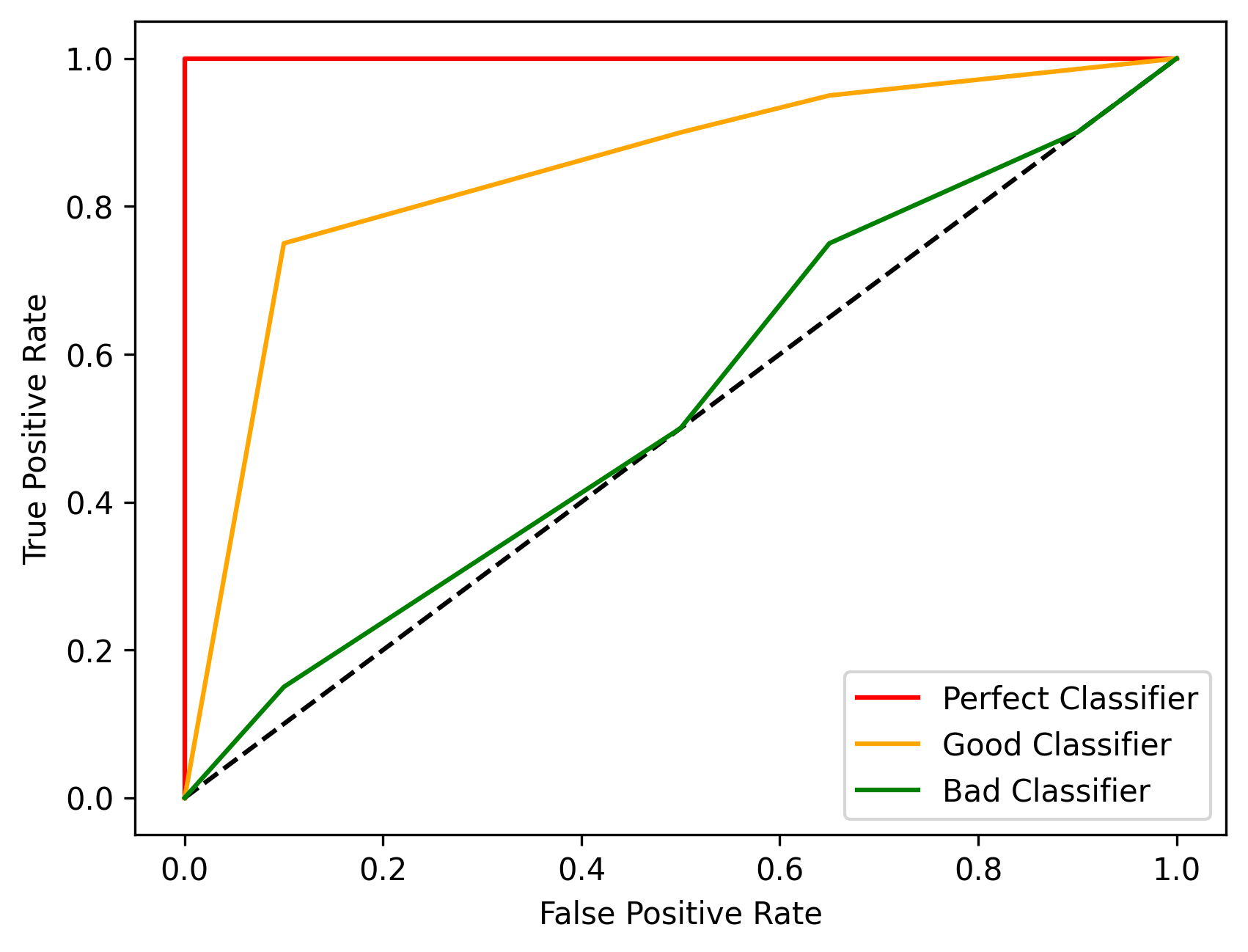# ROC Curve

The Receiver Operating Characteristic (ROC) curve evaluates the model performances at different threshold values by considering two parameters, the true positive rate and the false positive rate, which are defined as:

`true_positive_rate = tp/(tp + fn) = recall`
`false_positive_rate = fp/(fp + tn)`### Example

``````from odin.classes import Curves

my_curve = Curves.ROC_CURVE
# use my_curve as 'curve' parameter in the analyses
``````